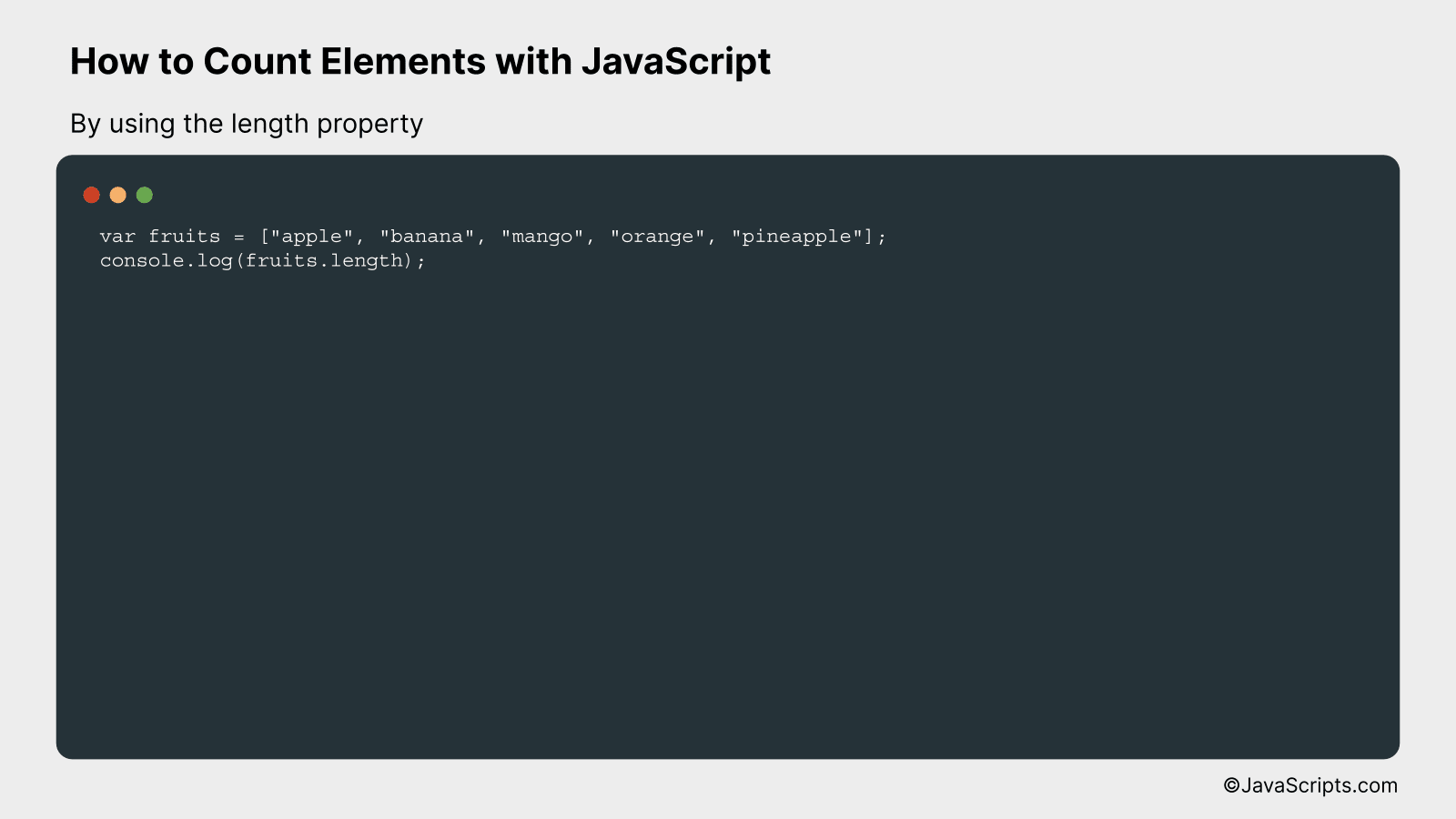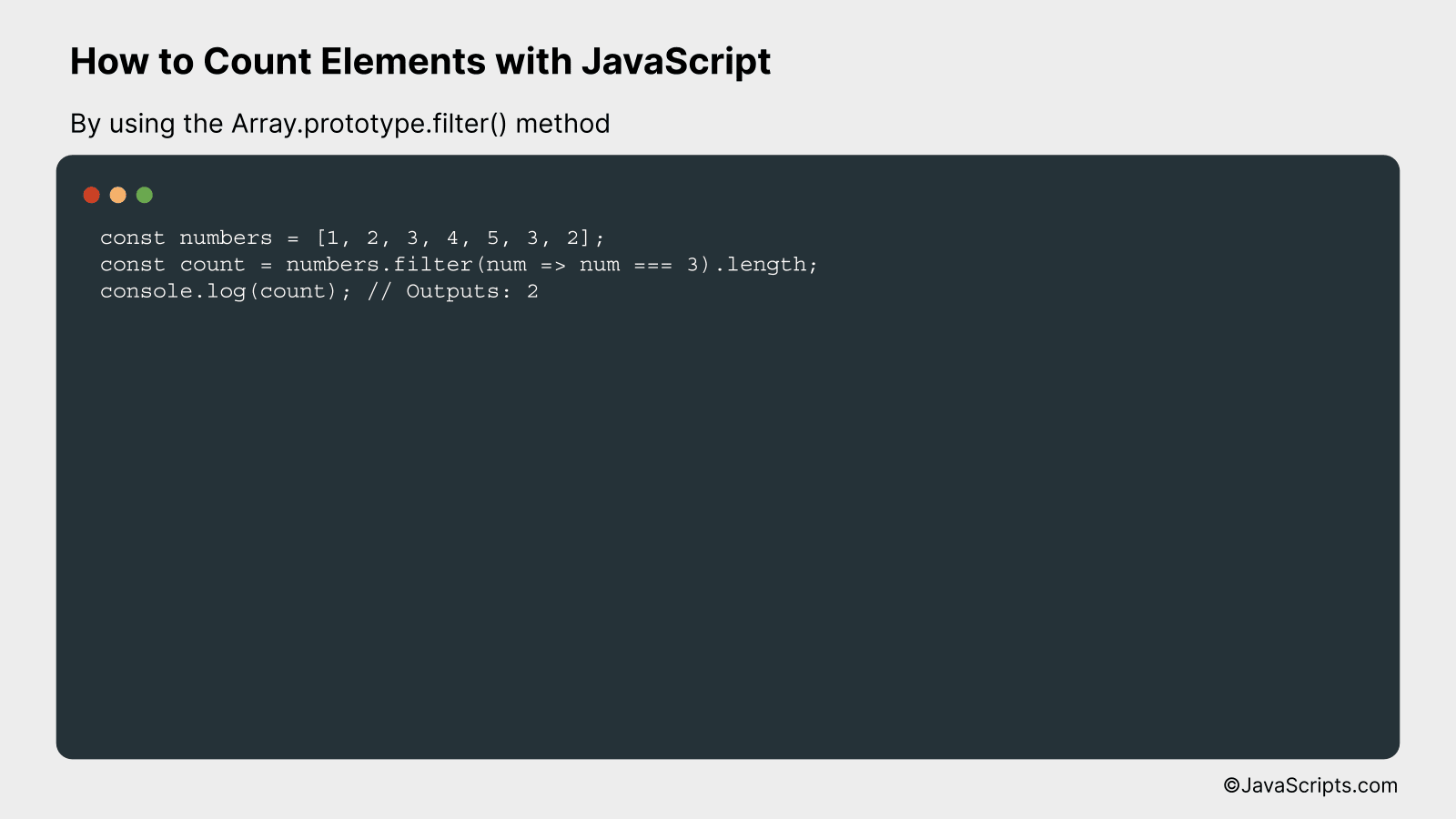# How to Count Elements with JavaScript

Ever wondered how to tally up elements using JavaScript? Well, you’re certainly not alone. There are countless situations where you might need to count elements in an array, track the number of times a specific value appears, or even just calculate the total number of elements in an object.

Good news! JavaScript offers a multitude of methods to achieve these tasks. Whether you’re a seasoned coder looking to brush up on your skills, or a novice eager to learn, we’ll tackle this topic together. Let’s dive into the ins and outs of counting elements in JavaScript, using clear, straightforward language.

In this post, we will be looking at the following 3 ways to count elements with JavaScript:

• By using the length property
• By using the Array.prototype.reduce() method
• By using the Array.prototype.filter() method

Let’s explore each…

## #1 – By using the length propertyThe ‘length’ property in JavaScript allows you to count the number of elements in an array or characters in a string. By referencing ‘.length’ after an array or string variable, the output will be the total elements or characters. For example, if we have an array named ‘fruits’, we can get the count of its elements by writing ‘fruits.length’.

``````
var fruits = ["apple", "banana", "mango", "orange", "pineapple"];
console.log(fruits.length);
```
```

### How it works

In JavaScript, arrays and strings are objects that have a property named ‘length’. This property returns the number of elements in an array or characters in a string. In our code, we declared an array ‘fruits’ with five elements. When we call ‘fruits.length’, it returns the number of elements in the ‘fruits’ array, which is 5.

• Step 1: We declare an array named ‘fruits’ with five elements ([“apple”, “banana”, “mango”, “orange”, “pineapple”]).
• Step 2: The ‘length’ property is a built-in property of arrays in JavaScript, which stores the number of elements in the array.
• Step 3: When we call ‘fruits.length’, JavaScript internally looks for the ‘length property’ of the ‘fruits’ array and returns its value.
• Step 4: The console.log function is used to print the output. It gets the returned value from ‘fruits.length’ which is 5, and prints it in the console.

## #2 – By using the Array.prototype.reduce() methodThe Array.prototype.reduce() method in JavaScript is utilized to count the frequency of elements in an array, transforming it into an object where keys are the unique elements and values are their corresponding counts.

Consider an example: If you have an array like `[1,2,2,3,3,3]`, you want to transform it into an object like `{'1':1, '2':2, '3':3}`, where ‘1’, ‘2’, and ‘3’ are the elements from the array and 1, 2, 3 are their counts respectively.

``````
let arr = [1,2,2,3,3,3];
let counts = arr.reduce((acc, val) => {
acc[val] = (acc[val] || 0) + 1;
return acc;
}, {});
``````

### How it works

The JavaScript reduce() method processes each element in the array one by one, invoking the provided function. The function takes an accumulator (acc) and the current value (val) as parameters. The accumulator is a value that’s carried over and modified on each iteration of the reduce method, and the current value is the currently processed element in the array.

• First, we define our array `arr`.
• We call reduce method on this array. This method takes two arguments: a callback function and an initial value for the accumulator. Here, the initial value of accumulator is an empty object `{}`.
• The callback function also takes two arguments: the accumulator `acc` (which is the object we are forming), and the current element `val` in the array.
• In the body of the callback function, we increment the count of the current element in the accumulator object. If the current element is not already a key in the accumulator object, it’s added with a value of 1. If it’s already a key, its value is incremented by 1.
• The accumulator object is returned at the end of each iteration and becomes the input accumulator for the next iteration.
• Finally, after all elements have been processed by the reduce method, we get the desired object where keys are the unique elements from the array and values are their counts.

## #3 – By using the Array.prototype.filter() methodIn JavaScript, you can use the Array.prototype.filter() method to count the number of elements in an array that meet a certain condition. This method creates a new array with all elements that pass the test implemented by the provided function. In this context, we’ll use it to count the elements instead by checking the length of the returned array.

``````
const numbers = [1, 2, 3, 4, 5, 3, 2];
const count = numbers.filter(num => num === 3).length;
console.log(count); // Outputs: 2
``````

### How it works

The Array.prototype.filter() will loop over each element in the array and apply the provided function, ‘num => num === 3’. If an element makes the function return true, it’s included in the new array. Then, by using the length property of the resulting array, we can count the number of elements that meet the condition.

• The filter() method takes a function as an argument, which will be applied to each element in the array.
• The function ‘num => num === 3’ returns true for the elements which are equal to 3, and false for the rest.
• The filter() method creates a new array with the elements that made the function return true.
• Finally, the length property of the new array gives us the count of how many elements are equal to 3 in the original array.

Related:

So, we’ve learned that counting elements with JavaScript is a fairly straightforward process. Whether we’re using a basic for loop, the forEach method, or the reduce function, each offers its own unique way of accomplishing the task.

To conclude, mastering these techniques will greatly enhance your ability to manipulate data in JavaScript. With practice and patience, you’ll be able to handle any counting challenge that comes your way!